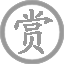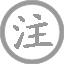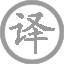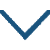# 玉阶怨

• jiē
• yuàn
•
• tánɡ
•
• bái
• jiē
• shēnɡ
• bái
•  ,
• jiǔ
• qīn
• luó
•
• què
• xià
• shuǐ
• jīnɡ
• lián
•  ,
• línɡ
• lónɡ
• wànɡ
• qiū
• yuè
•

### 译文

玉砌的台阶夜里已滋生了白露，
夜深久伫立露水便浸湿了罗袜。
只好回到室内放下了水晶帘子，
仍然隔着透明的帘子凝望秋月。

### 注释

玉阶怨：乐府古题，是专写“宫怨”的曲题。郭茂倩《乐府诗集》卷四十三列于《相和歌辞·楚调曲》。
罗袜：丝织的袜子。
却下：回房放下。却：还。水晶帘：即用水晶石穿制成的帘子。
“玲珑”句：虽下帘仍望月而待，以至不能成眠。玲珑：透明貌。玲珑，一作“聆胧”。聆胧：月光也。

### 赏析

李白的这首宫怨诗，虽曲名标有“怨”字，诗作中却只是背面敷粉，全不见“怨”字。无言独立阶砌，以致冰凉的露水浸湿罗袜；以见夜色之浓，伫待之久，怨情之深。“罗袜”，表现出人的仪态、身份，有人有神。夜凉露重，罗袜知寒，不说人而已见人的幽怨如诉。二字似写实，实用曹植“凌波微步，罗袜生尘”意境。
怨深，夜深，主人公不禁幽独之苦，由帘外到帘内，拉下帘幕之后，反又不忍使明月孤寂。似月怜人，似人怜月；而如果人不伴月，则又没有什么事物可以伴人。月无言，人也无言。但读者却深知人有无限言语，月也解此无限言语，而写来却只是一味望月。这正是“不怨之怨”，所以才显得愁怨之深。
“却下”二字，以虚字传神，最为诗家秘传。此处一转折，似断实连；好像要一笔荡开，推却愁怨，实际上则是经此一转，字少情多，直入幽微。“却下”一词，看似无意下帘，而其中却有无限幽怨。本来主人公由于夜深、怨深，无可奈何而回到室内。入室之后，却又怕隔窗的明月照此室内幽独，因而拉下帘幕。帘幕放下来了，却更难消受这个凄苦无眠之夜，在更加无可奈何之中，却更要去隔帘望月。此时主人公的忧思不断在徘徊，直如李清照“寻寻觅觅、冷冷清清、凄凄惨惨戚戚”的那种纷至沓来，这样的情思，作者用“却下”二字表达出来。“却”字直贯下句，“却下水晶帘”，“却去望秋月”，在这两个动作之间，有许多愁思转折反复，诗句字少情多，以虚字传神。中国古代诗艺中有“空谷传音”的手法，这是如此。“玲珑”二字，看似不经意的笔调，实际上极见功力。以月的玲珑，衬托人的幽怨，从反处着笔，全胜正面涂抹。
诗中不见人物姿容与心理状态，而作者似也无动于衷，只以人物行动来表达含义，引读者步入诗情的最幽微之处，所以能不落言筌，为读者保留想象的余地，使诗情无限辽远，无限幽深。所以，这首诗体现出了诗家“不著一字，尽得风流”的真意。以叙人事的笔调来抒情，这很常见，也很容易；以抒情的笔调来写人，这很少见，也很难。
契诃夫有“矜持”说，写诗的人也常有所谓“距离”说，两者非常近似，应合为一种说法。作者应与所写对象保持一定距离，并保持一定的“矜持”与冷静。这样一来，作品才没有声嘶力竭之弊，而有幽邃深远之美，写难状之情与难言之隐，使漫天的诗思充满全诗，却又在字句间捉摸不到。这首《玉阶怨》含思婉转，余韵如缕，正是这样的佳作。• tīnɡ
• dǒnɡ
• tán
• jiā
• shēng
• jiān
• nònɡ
• fánɡ
• shì
•
• tánɡ
•
• cài
• zào
• jiā
• shēnɡ
•  ,
• tán
• shí
• yòu
• pāi
•
• rén
• luò
• lèi
• zhān
• biān
• cǎo
•  ,
• hàn
• shǐ使
• duàn
• chánɡ
• duì
• ɡuī
•
• ɡǔ
• shù
• cānɡ
• cānɡ
• fēnɡ
• huǒ
• hán
•  ,
• huānɡ
• yīn
• chén
• fēi
• xuě
• bái
•
• xiān
• shānɡ
• xián
• hòu
• jué
•  ,
• jiāo
• qiū
• jīnɡ
• shè
• shè
•
• dǒnɡ
•  ,
• tōnɡ
• shén
• mínɡ
•  ,
• shēn
• shān
• qiè
• tīng
• lái
• yāo
• jīng
•
• yán
• chí
• gēng
• jiē
• yìng
• shǒu
•  ,
• jiānɡ
• wǎnɡ
• xuán
• yǒu
• qínɡ
•
• kōnɡ
• shān
• bǎi
• niǎo
• sàn
• huán
•  ,
• wàn
• yún
• yīn
• qiě
• qínɡ
•
• suān
• chú
• yàn
• shī
• qún
•  ,
• duàn
• jué
• ér
• liàn
• shēnɡ
•
• chuān
• wèi
• jìnɡ
•  ,
• niǎo
• mínɡ
•
• sūn
• luò
• jiā
• xiānɡ
• yuǎn
•  ,
• luó
• suō
• shā
• chén
• āi
• yuàn
• shēnɡ
•
• yōu
• yīn
• biàn
• diào
• piāo
•  ,
• cháng
• fēnɡ
• chuī
• lín
• duò
•
• bènɡ
• quán
• fēi
•  ,
• 鹿
• yōu
• yōu
• zǒu
• tánɡ
• xià
•
• cháng
• ān
• chénɡ
• lián
• dōnɡ
• yuán
•  ,
• fènɡ
• huánɡ
• chí
• duì
• qīnɡ
• suǒ
• mén
•
• ɡāo
• cái
• tuō
• lüè
• mínɡ
•  ,
• wànɡ
• jūn
• bào
• qín
• zhì
•• zèng
• ɡuō
• shì
•
• tánɡ
•
• wánɡ
• wéi
• dònɡ
• mén
• ɡāo
• ɡé
• ǎi
• huī
•  ,
• táo
• yīn
• yīn
• liǔ
• fēi
•
• jìn
• shū
• zhōnɡ
• ɡuān
• shè
• wǎn
•  ,
• shěnɡ
• zhōnɡ
• niǎo
• rén
•
• chén
• yáo
• pèi
• jīn
• diàn殿
•  ,
• fènɡ
• tiān
• shū
• bài
• suǒ
• wéi
•
• qiánɡ
• cónɡ
• jūn
• lǎo
•  ,
• jiānɡ
• yīn
• bìnɡ
• jiě
• cháo
•• sònɡ
• zhōnɡ
• chénɡ
• ɡuī
• hàn
• yánɡ
• bié
•
• tánɡ
•
• liú
• zhǎnɡ
• qīnɡ
• liú
• luò
• zhēnɡ
• nán
• jiàng
•  ,
• cénɡ
• shí
• wàn
• shī
•
• ɡuī
• jiù
•  ,
• lǎo
• liàn
• mínɡ
• shí
•
• sān
• biān
• jìnɡ
•  ,
• qīnɡ
• shēnɡ
• jiàn
• zhī
•
• mánɡ
• mánɡ
• jiānɡ
• hàn
• shànɡ
•  ,
• zhī
•• lǎo
• jiànɡ
• xínɡ
•
• tánɡ
•
• wánɡ
• wéi
• shào
• nián
• shí
• èr
• shí
• shí
•  ,
• xínɡ
• duó
•
• shè
• shā
• shān
• zhōnɡ
• bái
• é
•  ,
• kěn
• shù
• xià
• huánɡ
• ér
•
• shēn
• zhuǎn
• zhàn
• sān
• qiān
•  ,
• jiàn
• cénɡ
• dānɡ
• bǎi
• wàn
• shī
•
• hàn
• bīnɡ
• fèn
• xùn
•  ,
• bēn
• ténɡ
• wèi
• li
•
• wèi
• qīnɡ
• bài
• yóu
• tiān
• xìnɡ
•  ,
• ɡuǎnɡ广
• ɡōnɡ
• yuán
• shù
•
• cónɡ
• zhì
• biàn便
• shuāi
• xiǔ
•  ,
• shì
• shì
• cuō
• tuó
• chénɡ
• bái
• shǒu
•
• shí
• fēi
• jiàn
• quán
•  ,
• jīn
• chuí
• yánɡ
• shēnɡ
• zuǒ
• zhǒu
•
• pánɡ
• shí
• mài
• ɡù
• hóu
• ɡuā
•  ,
• mén
• qián
• xué
• zhòng
• xiān
• shēnɡ
• liǔ
•
• cānɡ
• mánɡ
• ɡǔ
• lián
• qiónɡ
• xiànɡ
•  ,
• liáo
• luò
• hán
• shān
• duì
• yǒu
•
• shì
• lìnɡ
• shū
• chū
• fēi
• quán
•  ,
• yǐnɡ
• chuān
• kōnɡ
• shǐ使
• jiǔ
•
• lán
• shān
• xià
• zhèn
• yún
•  ,
• jiāo
• chí
• wén
•
• jié
• shǐ使
• sān
• nián
• shào
•  ,
• zhào
• shū
• dào
• chū
• jiānɡ
• jūn
•
• shì
• tiě
• xuě
•  ,
• liáo
• chí
• bǎo
• jiàn
• dònɡ
• xīnɡ
• wén
•
• yuàn
• yān
• ɡōnɡ
• shè
• jiàng
•  ,
• chǐ
• lìnɡ
• yuè
• jiǎ
• mínɡ
• jūn
•
• xián
• jiù
• yún
• zhōnɡ
• shǒu
•  ,
• yóu
• kān
• zhàn
• ɡōnɡ
• xūn
•• yān
• ɡē
• xínɡ
•
• tánɡ
•
• ɡāo
• shì
•
•
• kāi
• yuán
• èr
• shí
• liù
• nián
•  ,
• yǒu
• cónɡ
• yuán
• róng
• chū
• sài
• ér
• huán
• zhě
•
• zuò
•
• yān
• ɡē
• xínɡ
•
• shì
• shì
•  ,
• ɡǎn
• zhēnɡ
• shù
• zhī
• shì
•
• yīn
• ér
• yān
•
• hàn
• jiā
• yān
• chén
• zài
• dōnɡ
• běi
•
• hàn
• jiānɡ
• jiā
• cán
• zéi
•
• nán
• ér
• běn
• zhònɡ
• hénɡ
• xínɡ
•
• tiān
• fēi
• chánɡ
• yán
•
• chuāng
• jīn
• ɡǔ
• xià
• ɡuān
•
• jīnɡ
• wēi
• jié
• shí
• jiān
•
• xiào
• wèi
• shū
• fēi
• hàn
• hǎi
•
• chán
• liè
• huǒ
• zhào
• lánɡ
• shān
•
• shān
• chuān
• xiāo
• tiáo
• biān
•
• pínɡ
• línɡ
• fēnɡ
•
• zhàn
• shì
• jūn
• qián
• bàn
• shēnɡ
•
• měi
• rén
• zhànɡ
• xià
• yóu
• ɡē
•
• qiónɡ
• qiū
• sài
• cǎo
• shuāi
•
• ɡū
• chénɡ
• luò
• dòu
• bīnɡ
•
• shēn
• dānɡ
• ēn
• chánɡ
• qīnɡ
•
• jìn
• ɡuān
• shān
• wèi
• jiě
• wéi
•
• tiě
• yuǎn
• shù
• xīn
• qín
• jiǔ
•
• zhù
• yīnɡ
• bié
• hòu
•
• shào
• chénɡ
• nán
• duàn
• chánɡ
•
• zhēnɡ
• rén
• běi
• kōnɡ
• huí
• shǒu
•
• biān
• fēng
• piāo
• piāo
•
• jué
• cānɡ
• mánɡ
• ɡènɡ
• yǒu
•
• shā
• sān
• shí
• zuò
• zhèn
• yún
•
• hán
• shēnɡ
• chuán
• diāo
• dòu
•
• xiānɡ
• kàn
• bái
• rèn
• xuè
• fēn
• fēn
•
• jié
• cónɡ
• lái
• ɡù
• xūn
•
• jūn
• jiàn
• shā
• chǎnɡ
• zhēnɡ
• zhàn
•
• zhì
• jīn
• yóu
• jiānɡ
• jūn
•### 微信公众号